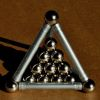#### You may also like### Tetra Inequalities

Can you prove that in every tetrahedron there is a vertex where the three edges meeting at that vertex have lengths which could be the sides of a triangle?### Staircase

Solving the equation x^3 = 3 is easy but what about solving equations with a 'staircase' of powers?### Proof Sorter - the Square Root of 2 Is Irrational

Try this interactivity to familiarise yourself with the proof that the square root of 2 is irrational. Sort the steps of the proof into the correct order.

# Be Reasonable

##### Age 16 to 18Challenge Level

Thank you to M. Grender-Jones for this solution.

To show that $\sqrt{2}$, $\sqrt{3}$ and $\sqrt{5}$ cannot form part of any arithmetic progression we give a proof by contradiction. Suppose they can, then $$\sqrt 3- \sqrt 2 = px$$ $$\sqrt 5 - \sqrt 2 = qx$$ where $x$ is the common difference of the progression, and $p$ and $q$ are integers.

Eliminating $x$ from these two equations we get $$p(\sqrt 5 - \sqrt 2) = q(\sqrt 3 - \sqrt 2)$$ so $$q\sqrt 3 = p\sqrt 5 + (q-p)\sqrt 2.$$ We know $p$ and $q$ are integers so to simplify this expression write $p-q = s$ where $s$ is an integer: $$q\sqrt 3 = p\sqrt 5 + s\sqrt 2.$$ Squaring this: $$3q^2 = 5p^2 + 2s^2 + 2ps\sqrt 10.$$ Rearranging this expression gives: $$\sqrt 10 = {3q^2 - 5p^2 - 2s^2 \over 2ps}.$$ As $\sqrt 10$ is irrational and all the other terms in this expression are integers this is impossible and we have reached a contradiction. Therefore our assumption was false and $\sqrt 2$, $\sqrt 3$ and $\sqrt 5$ cannot be terms of an AP.

By the same method can you prove that $\sqrt{1}$, $\sqrt{2}$ and $\sqrt{3}$ cannot be terms of ANY arithmetic progression?